## Table of ContentOpen Access

ARTICLE

# Exploiting the Direct Link in IRS Assisted NOMA Networks with Hardware Impairments

Ziwei Liu1, Xinwei Yue1,*, Shuo Chen1, Xuliang Liu2, Yafei Wang1, Wanwei Tang3

1 Key Laboratory of Modern Measurement & Control Technology, Ministry of Education, School of Information and Communication Engineering, Beijing Information Science and Technology University, Beijing, 100101, China
2 The Party Committee Office, Yellow River Conservancy Technical Institute, Kaifeng, 475001, China
3 Tangshan University, Tangshan, 063000, China

* Corresponding Author: Xinwei Yue. Email:(This article belongs to this Special Issue: Recent Advances in Backscatter and Intelligent Reflecting Surface Communications for 6G-enabled Internet of Things Networks)

Computer Modeling in Engineering & Sciences 2023, 136(1), 767-785. https://doi.org/10.32604/cmes.2023.025300

## Abstract

Hardware impairments (HI) are always present in low-cost wireless devices. This paper investigates the outage behaviors of intelligent reflecting surface (IRS) assisted non-orthogonal multiple access (NOMA) networks by taking into account the impact of HI. Specifically, we derive the approximate and asymptotic expressions of the outage probability for the IRS-NOMA-HI networks. Based on the asymptotic results, the diversity orders under perfect self-interference cancellation and imperfect self-interference cancellation scenarios are obtained to evaluate the performance of the considered network. In addition, the system throughput of IRS-NOMA-HI is discussed in delay-limited mode. The obtained results are provided to verify the accuracy of the theoretical analyses and reveal that: 1) The outage performance and system throughput for IRS-NOMA-HI outperforms that of the IRS-assisted orthogonal multiple access-HI (IRS-OMA-HI) networks; 2) The number of IRS elements, the pass loss factors, the Rician factors, and the value of HI are pivotal to enhancing the performance of IRS-NOMA-HI networks; and 3) It is recommended that effective methods of reducing HI should be used to ensure system performance, in addition to self-interference cancellation techniques.

## Keywords

1  Introduction

As a promising technology to achieve significant improvement in system throughput and energy efficiency, non-orthogonal multiple access (NOMA) has recently attracted growing attention for the sixth-generation (6G) communication networks [1,2]. Different from the traditional orthogonal multiple access (OMA), the main advantage of NOMA technology is that it allows multiple users to utilize the same resource and realize the correct demodulation by introducing the successive interference cancellation (SIC) technology, which can improve the utilization of spectrum resources . It has been demonstrated that power domain NOMA can ensure much higher throughput in underlay cognitive radio networks than conventional OMA . NOMA also can contribute significantly to improving the quality of service, which provides a balanced service comparatively for serving multiple devices [5,6]. In order to enhance the system’s performance, the integration of NOMA and cooperative communication has been proposed in [7,8]. In particular, the authors of  concluded that the outage behaviors and ergodic rate of cooperative NOMA systems are better compared to traditional cooperative communication. To achieve enhanced spectrum efficiency, the authors of [10,11] analyzed the outage performance of users for amplify-and-forward and decode-and-forward relays in cooperative NOMA systems.

Meanwhile, intelligent reflecting surface (IRS) is deemed as another new transmission technology for future wireless networks , which consists of numerous precisely designed arrangements of electromagnetic units, and the signal is controlled by changing the phase and amplitude of the incident wave . There are some advantages of IRS: i) IRS can enrich the channel scattering conditions, enhance the system’s multiplexing gain, and improve the received signal strength ; ii) The lightweight and low power requirements of the IRS make it easy to deploy on various scattering surfaces in wireless propagation environment, such as buildings, roadside billboards, windows, and interior walls ; and iii) Unlike the traditional active relay, IRS reflects signals in full-duplex mode through passive beamforming and does not impose additional energy consumption and noise . These features have attracted researchers to apply it to various new wireless communication scenarios. Specifically, the authors of  showed that IRS deployment could effectively improve wireless networks’ throughput, energy efficiency and coverage performance. In , the IRS-assisted wireless communications were introduced in an end-to-end transmission scenario, which demonstrated that deploying an IRS structure can substantially improve the pairwise error probability metric. The aforementioned works manifest that IRS yields significant performance in communication scenarios.

Inspired by the appealing advantages of IRS and NOMA, some recent works have investigated the interplay between IRS and NOMA, which can achieve enhanced massive connectivity with higher network spectral efficiency and user fairness . The authors of  demonstrated that combining IRS and NOMA is an effective strategy to enhance communication coverage and energy efficiency. Furthermore, the authors of  proved that IRS-NOMA could achieve superior performance compared to the counterpart IRS-OMA schemes. With the help of a 1-bit coding scheme, the outage probability and ergodic rate for IRS-NOMA networks under Rayleigh fading channels have been analyzed considering the impact of perfect SIC (pSIC) and imperfect SIC (ipSIC) scenarios . Moreover, in , the authors assessed the impact of IRS phase-shifting design on the outage probability through two-phase configurations, i.e., a random phase and coherent phase shifting. The authors of  minimized the transmit power of the IRS-NOMA system by optimizing the beamforming vector at base station (BS) and the phase shifters at IRS. As a further development, the interplay between IRS and NOMA is widely investigated for various technologies like unmanned aerial vehicle , deep reinforcement learning , mobile edge computing  and physical layer security . The previous works were applied to the ideal hardware conditions.

However, it is challenging to achieve the perfect performance in practice with the existence of hardware impairments (HI), and residual interference [33,34]. Specifically, HI causes errors in the transmitted signal at the transmitter and reduces the detection quality of the received signal at the receiver . Under the realistic assumptions of residual HI, channel estimation errors and ipSIC, the authors of  investigated the NOMA systems with an emphasis on reliability and security. In , the performance of full-duplex NOMA networks over Rician fading channels was described and shown the influence of ipSIC on the system is more pronounced than that of HI. The authors employed multiple relays with finite energy storage capability to assist the BS to two NOMA device users’ transmission transmission of the NOMA network in , and the outage probability expressions of users were derived to evaluate the system performance. Considering the IRS-assisted wireless communications, the existence of HI will affect both the source transmit beamforming and the IRS reflecting beamforming designs . To compensate for the losses caused by HI, various analog and digital signal processing schemes are discussed in . Despite these efforts, the HI still degrades the performance of wireless communication networks. As a further advance, the authors of [41,42] analyzed the system performance under the impact of HI at both BS and users on various NOMA and multiple input multiple output (MIMO) networks.

1.1 Motivations and Contributions

Motivated by the aforementioned observations, we study the performance of an IRS-NOMA network by incorporating signal distortions caused by HI. Until now, most of the existing research contributions for IRS-NOMA with ipSIC/pSIC over Rician fading channels have considered the optimization questions. Motivated by the observations mentioned earlier [43,44], we considered an IRS-NOMA communication system over Rician channels by incorporating signal distortions caused by HI in the transmitter and receiver. In contrast to the literature , we extend our analytical results from the Rayleigh channels to the more general Rician fading channels. Compared to , the direct communication link from the BS to the users is taken into account in this paper. This is another motivation to develop this treatise. More specifically, we investigate the performance of paired users, i.e., the user n and user f for IRS-NOMA-HI networks, in terms of outage probability and system throughput in delay-limited transmission mode. Moreover, the collective impact of HI and residual interference is also considered in the considered networks. IRS-OMA-HI is shown as a comparison benchmark for better evaluation of system performance. Against the previous works, the contributions of the current paper can be summarized as follows:

1.    We derive the expressions of outage probability for the user n with ipSIC/pSIC in IRS-NOMA-HI networks over Rician fading channels. To gain the corresponding diversity order, we also derive the asymptotic outage probability expressions in the high SNR region and obtain the diversity orders of the user n with ipSIC/pSIC schemes. We observe an error floor for the outage probability of the user n with ipSIC in the high SNR regime.

2.    We derive the expressions of outage probability for the user f in IRS-NOMA-HI networks. Based on the asymptotic results, the diversity orders are obtained. We further confirm that the diversity order is connected with the number of IRS elements and the Rician factor K. We also derive the exact expressions of outage probability for IRS-OMA-HI.

3.    We evaluate the system throughput of IRS-NOMA-HI and IRS-OMA-HI networks in delay-limited transmission modes. We further compare the outage behaviors and system throughput of users for IRS-NOMA-HI with IRS-OMA-HI. Through extensive simulation results, it is shown that the outage performance and system throughput of IRS-NOMA-HI is superior to that of IRS-OMA-HI.

1.2 Organization

The remainder of this paper is structured as follows. In Section 2, the system model for IRS-NOMA-HI is introduced in detail. The expressions of outage probability are derived and the system throughput is studied in Section 3. Furthermore, Section 4 gives simulation results and corresponding performance analysis, followed by conclusions and future directions in Section 5.

2  System Model

We consider an IRS-NOMA-HI network, as depicted in Fig. 1, where one BS communicates with two users via the assistance of an IRS. The two users are classified as the nearby user n and the distant user f, which can receive both the superimposed signal from the BS and the signal reflected by IRS. To simplify the design, the BS and users are equipped with a single antenna while an IRS consists of M configurable elements. And Φ=diag(ejθ1,,ejθm,,ejθM) denote the phase shifting matrixes, where θm[0,2π) is the phase shift of the m-th reflection element.1 All wireless communication channels for the IRS-NOMA-HI networks are assumed to follow the baseband equivalent Rician fading channel models. Note that the channel coefficients from the BS to users, from BS to IRS, and from IRS to users are denoted by hsi, hsrM×1 and hriM×1 with i(n,f), respectively.2 More specifically, let hsi=αsi(KK+1+1K+1h~si), αsi=ηdsi, and h~siCN(0,1), where αsi denotes the large-scale fading coefficients. dsi, ∂ and η denote the distance from BS to the user i, path loss exponent and frequency-dependent factor, respectively. The vectors of channel coefficients from the BS to IRS and from the IRS to users are given as hsr=[hsr1⋅ ⋅ ⋅hsrmhsrM ]H and hri=[ hri1⋅ ⋅ ⋅hrimhriM ]H , where hιm=αιm(KK+1+1K+1h~ιm) and hιmCN(0,1) with ι(sr,ri). The Rician factor K is the ratio of the power of the direct path signal to the variance of the other multipath power, which reflects the influence of multipath scattering on signal distribution. In particular, the power allocation for the user n and the user f are an and af, satisfying the relationship an + af = 1 and an < af. The coherent phase shifting has the ability to improve the performance of IRS-NOMA-HI network, where the phase shift of each reflecting element is matched with the phases of its incoming and outgoing fading channels. This paper chooses coherent phase shifting to simplify computational complexity and provide distinct analytical results. It is worth pointing out that the random phase-shifting of IRS can also affect the outage behaviors, which will be set aside in our future work. To characterize the optimal performance of the IRS-NOMA-HI networks, we assume that the IRS can obtain perfect channel state information of the users.Figure 1: System model for IRS-NOMA-HI networks

2.1 IRS-NOMA-HI

The BS simultaneously transmits the signals of two users by adopting superposition coding, and the user n receives both the superimposed signal from the BS and the signal reflected by IRS. Accordingly, the received signal is expressed by

yn=(hsn+hsrHΦhrn)​​(​​anPsxf+afPsxn)​​+w+nn,(1)

where Ps denotes the normalized transmission power, nn denotes the complex additive white Gaussian noise (AWGN) sample with the mean power N0 at user n, and w is HI term and can be modeled by a complex Gaussian distributed random variable with zero mean and finite variance, w(0,(kT2+kR2)Ps). It is worth noting that kT and kR represent the levels of HI at the transmitter and the receiver, respectively. Especially, they are modeled as a complex Gaussian process with zero mean and variances |(hsn+hsrHΦhrn)|2kT2Ps, |(hsn+hsrHΦhrn)|2kR2Ps, respectively.

According to the NOMA principle, SIC is adopted at user n to detect the information of user f. Therefore, the received signal-to-interference-plus-noise ratio (SINR) at the user n for detecting xf is given by

γnf=| hsn+hsrHΦhrn |2ρsaf| hsn+hsrHΦhrn |2ρsan+| hsn+hsrHΦhrn |2​​(kT2+kR2)ρs+1, (2)

where ρs=PsN0 is the transmit SNR. After information xf is detected, it is eliminated from the received signal by performing the SIC process. Thus, the SINR at user n for detecting xn is given by

γn=| hsn+hsrHΦhrn |2ρsan| hsn+hsrHΦhrn |2(kT2+kR2)ρs+ϖ| hI |2ρs+1, (3)

where ϖ=0and 0<ϖ1 denotes the situations of pSIC and ipSIC. Without loss of generality, the complex channel coefficient of residual interference from the ipSIC is denoted as hICN(0,ΩI).

With the help of IRS, the received signal at user n is given by

yf=(hsf+hsrHΦhrf)​​(anPsxn+afPsxf)​​+w+nf, (4)

where nf is the AWGN at user f with the mean power N0.

The received SINR to decode xf from user f can be given by

γf=| hsf+hsrHΦhrf |2ρsaf| hsf+hsrHΦhrf |2ρsan+| hsf+hsrHΦhrf |2​​(kT2+kR2)ρs+1. (5)

2.2 IRS-OMA-HI

In this subsection, the IRS-OMA-HI scheme is used as a benchmark for comparison with the NOMA scheme. The BS transmit a superimposed signal that propagates from the BS for the users through the IRS, the SINR at OMA users can be expressed as

γiOMA=| hsi+hsrHΦhri |2ρsai| hsi+hsrHΦhri |2(kT2+kR2)ρs+1. (6)

3  Outage Probability

Outage probability metrics are critical to the reliability of transmissions in 6G systems. Hence, this section evaluates the outage performance of the IRS-NOMA-HI network. Specifically, we derive the expressions of outage probability for each user in the pSIC and ipSIC scenarios. Then, we obtain the diversity orders to provide further insights into the communication performance.

3.1 Statistical Channel Characterization

In order to facilitate the derivation of outage probabilities, we characterize the statistical properties of the Rician channel in this subsection. Z=||hsi|+m=1M|hsrmhrim||2 is an random variables (RVs), where the cumulative distribution function (CDF) of Z should be obtained. Let |hsf| be an RV, with the probability density function (PDF) can be given by

f|hsi|(x)=2x(K+1)αsieKex2(K+1)αsiK0(2yK(K+1)αsi), (7)

where K0() is the modified Bessel function of the second kind with order zero.

The PDF of X=m=1M|hsrmhrnm| is more difficult to calculate. Based on the characteristics of the Rician fading channels, the PDF is obtained using the Laguerre series approximation, which can be approximated as

fX(x)1ϕkΓ(φk+1)(xϕk)φkexp[xϕk], (8)

where φk=Mμk2ΩK1 and ϕk=ΩKμk are defined through the mean μk and the variance ΩK. μk=παsrαrn4(K+1)[L12(K)]2, ΩK=αsrαrn{1π216(1+K)2[L12(K)]4}, and L12(K)=e12[(1K)K0(K2)KK1(K2)]. Based on (8) and (9), after some arithmetical manipulations, the CDF of Z can be written as

FZ(z)=0z1ϕkΓ(φk+1)(xϕk)φkexp[xϕk]×[1Q(2K,(zx)2(K+1)αsf)]dx. (9)

3.2 Outage Probability for IRS-NOMA-HI

In wireless communication networks, the theoretical analyses of outage probability are the crucial works, which can guide the design and performance optimization of wireless communication systems’ practical. In the next part, the outage behaviors of user n and user f for IRS-NOMA-HI networks are investigated in details. Furthermore, it is worth pointing out that implementing MRC in IRS-NOMA-HI transmission will further enhance the performance of IRS-NOMA-HI, which are set aside for our future work.

3.2.1 User n of Outage Probability

For the user n, system communication is interrupted when one of the following two events occurs. Case i) User n is unable to detect the signal xf of user f; Case ii) User n cannot decode its own signal xn after detecting xf. Thus, the outage probability of user n for RIS-NOMA-HI networks is expressed as

Pn=Pr(γnf<γthf)+Pr(γnf>γthf,γn<γthn), (10)

where γthf=2Rf1 and γthn=2Rn1 denote the SNR threshold of user n and user f. Specially, Rn and Rf is the target rate at the users to detect xn and xf. The outage probability expression of user n for the IRS-NOMA-HI isis approximated by the following theorem.

Theorem 1. The expression of outage probability for user f with ipSIC in IRS-NOMA-HI networks is approximated by

PnipSICΥr=1Rl=1LAr(1xl2)(xl+1)φν~φ+1exp[(xl+1)ν~2ϕ]{1Q(2K,[(1+xl)ν~2]2(K+1)αsn)}, (11)

where β=γthnρs[anγthn(kT2+kR2)], ν~=β(φkΩIxrρs+1), Υ=π(2ϕk)φk+1LΓ(φk+1), xl=cos(2l12Lπ), Ar=(R!)2xr[LP+1(xr)]2, xr and Ar are the abscissas and weight of Gauss-Laguerre quadrature, respectively.

Proof: See Appendix A.

Corollary 1. For the particular case ε=0, the expression of outage probability for user f with pSIC in IRS-NOMA-HI networks is approximated by

PnpSICl=1Lπβ(K+1)Al2LΓ(φk+1)αsne[β(K+1)(xl+1)24αsn+K]K0((xl+1)βK(K+1)αsn)γ(φk+1,ββxl2ϕ), (12)

where Al=(xl+1)1xl2 and γ(α,x)=0xettα1dt is the incomplete Gamma function. Similar to the proof of Theorem 1, substituting ε=0 into (A.1) and applying Gauss-Laguerre quadrature, the outage probability of user f with pSIC in IRS-NOMA-HI networks can be obtained.

3.2.2 User f of Outage Probability

Similarly, the outage event generates when user f fails to detect the signals, the outage probability of user f for IRS-NOMA-HI networks is expressed as

Pf=Pr(γf<γthf). (13)

Theorem 2. Based on (13), the expression of outage probability of user f can be approximated by

Pfl=1Lπα(K+1)Al2LαsfeKΓ(φ+1)exp(α(K+1)(xl+1)24αsf)K0((xl+1)αK(K+1)αsf)γ(φ+1,ααxl2ϕ), (14)

where α=γthfρs(afγthfan(kT2+kR2)), φ=Mμ2Ω1 and ϕ=Ωμ are defined through the mean μ and the variance Ω. Specifically, μ=παsrαrf4(K+1)[L12(K)]2 and Ω=αsrαrf{1π216(1+K)2[L12(K)]4}.

Proof: See Appendix B.

3.3 Outage Probability for IRS-OMA-HI

For IRS-OMA-HI networks, the outage of user f and user n are defined as the probability that the instantaneous SNR is below the threshold SNR γthiOMA. Thus, the outage probability of users in IRS-OMA-HI networks is denoted by

PiOMA=Pr[γiOMA<γthiOMA], (15)

where γthiOMA=2RiOMA1 with i(n,f). Similar to the proof of IRS-NOMA-HI networks, the outage probability in IRS-OMA-HI networks is approximated by

PiOMAl=1Lπλi(K+1)2LαsieKΓ(φ+1)e[λi(K+1)(xl+1)24αsi]AlK0((xl+1)λiK(K+1)αsi)γ(φ+1,λiλixl2ϕ), (16)

where λi=γthiOMAρs[aiγthiOMA(kT2+kR2)].

3.4 Diversity Analysis

To gain more insights, we select the diversity order to evaluate the outage behaviors in the high SINR region  based on the above analytical results. In the IRS-NOMA-HI networks, the diversity order is mathematically defined as

d=limρslog(P(ρs))logρs, (17)

where ρs is the transmit SNR, and P(ρs) denotes the asymptotic outage probability of the users in the high SNR regime for the considered IRS-NOMA-HI networks, which are summarized in the following corollaries.

Corollary 2. When ρs tends to infinity, β tends to γthnanγthn(kT2+kR2), the asymptotic outage probability of the user n with ipSIC in IRS-NOMA-HI networks is given by

Pn,ipSICΥr=1Rl=1LAr(1xl2)(xl+1)φνnφ+1e(xl+1)νn2ϕ{1Q(2K,[(1+xl)νn2]2(K+1)αsn)}, (18)

where νn=βnΩIxr, and βn=γthnanγthn(kT2+kR2).

Remark 1. Upon substituting (18) into (17), i.e., dnipSIC=limρslogPn,ipSIClogρs=0. Hence, a zero diversity order of user n with ipSIC for IRS-NOMA-HI can be obtained, which is caused by residual interference from ipSIC.

Corollary 3. Expanding the incomplete gamma function γ(φ+1,ββxl2ϕ) of (12) in terms of the series as n=0(1)nββxl2ϕφ+1+nn!(φ+1+n). When ρs tends to infinity, the asymptotic outage probability of the user f with pSIC in IRS-NOMA-HI networks is given by

Pn,pSIC=l=1Lπβ(K+1)Al2L(φk+1)Γ(φk+1)αsne[β(K+1)(xl+1)24αsn+K](ββxl2ϕk)φk+1K0((xl+1)βK(K+1)αsn). (19)

Remark 2. Upon substituting (19) into (17) and after some manipulations, the diversity order for the user f with pSIC in IRS-NOMA-HI is obtained as Mμk22ΩK+1. It can be observed that the diversity order of user f with pSIC is in connection with the number of IRS elements and Rician factor K.

Corollary 4. When ρs tends to infinity, the asymptotic outage probability of the user n in IRS-NOMA-HI networks is written by

Pf=l=1Lπα(K+1)Al2L(φ+1)Γ(φ+1)αsfe[α(K+1)(xl+1)24αsf+K](ααxl2ϕ)φ+1K0((xl+1)αK(K+1)αsf). (20)

Remark 3. Upon substituting (20) into (17), the diversity order of user n is equal to Mμ22Ω+1, which is also related to the number of IRS elements and Rician factor K.

Remark 4. Similar to the proof of the diversity order for IRS-NOMA-HI, the diversity order of user f and user n for IRS-OMA-HI are equal to Mμk22ΩK+1 and Mμ22Ω+1, respectively, which are also related to the number of IRS elements and Rician factor K.

We plotted the diversity order of IRS-NOMA-HI and IRS-OMA-HI networks in a table, as shown in Table 1.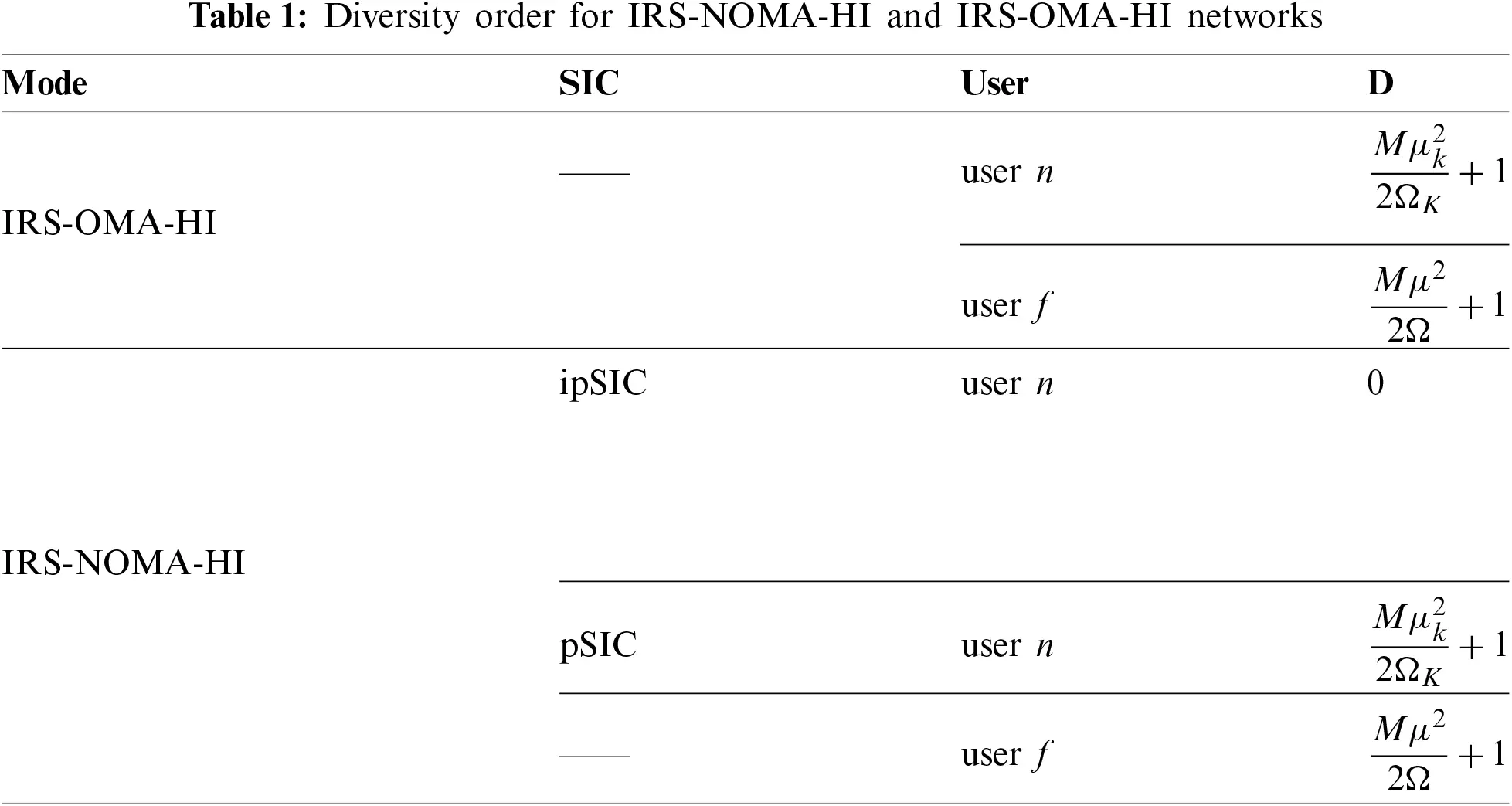3.5 Throughput Analysis

The system throughput metric is paramount to evaluating the reliability of the transmission in 6G systems. Next, by using the derived results of outage probability above, the delay-limited system throughput of IRS-NOMA-HI networks can be formulated by

Rδ=(1Pnδ)Rn+(1Pf)Rf, (21)

where δ(pSIC,ipSIC), PnipSIC, PnpSIC and Pf can be obtained from (11), (12) and (14), respectively.

4  Numerical Results

In this section, we aim to confirm through Monte-Carlo simulations the accuracy of our previous mathematical analysis and illustrate the outage performance of the IRS-NOMA-HI networks. To verify the feasibility of the IRS-NOMA-HI networks, IRS-OMA-HI is shown as the comparison benchmarks, and the performance of the transmission schemes is evaluated through computer simulation. The simulation results are averaged over 106 realizations. More specifically, the parameters adopted are presented in Table 2 to highlight the performance of the proposed scheme, which is similar to many NOMA research contributions in [10,26,27]. BPCU denotes the short for a bit per channel use and the unit of normalized distance d for dimensionless physical quantity is generally taken as one. Furthermore, we show the impact of residual interference, target rate, Rician factor and IRS elements on the performance of IRS-NOMA-HI networks. It is worth pointing out that these dots are the Monte Carlo simulation values, and the lines are the theoretically derived values.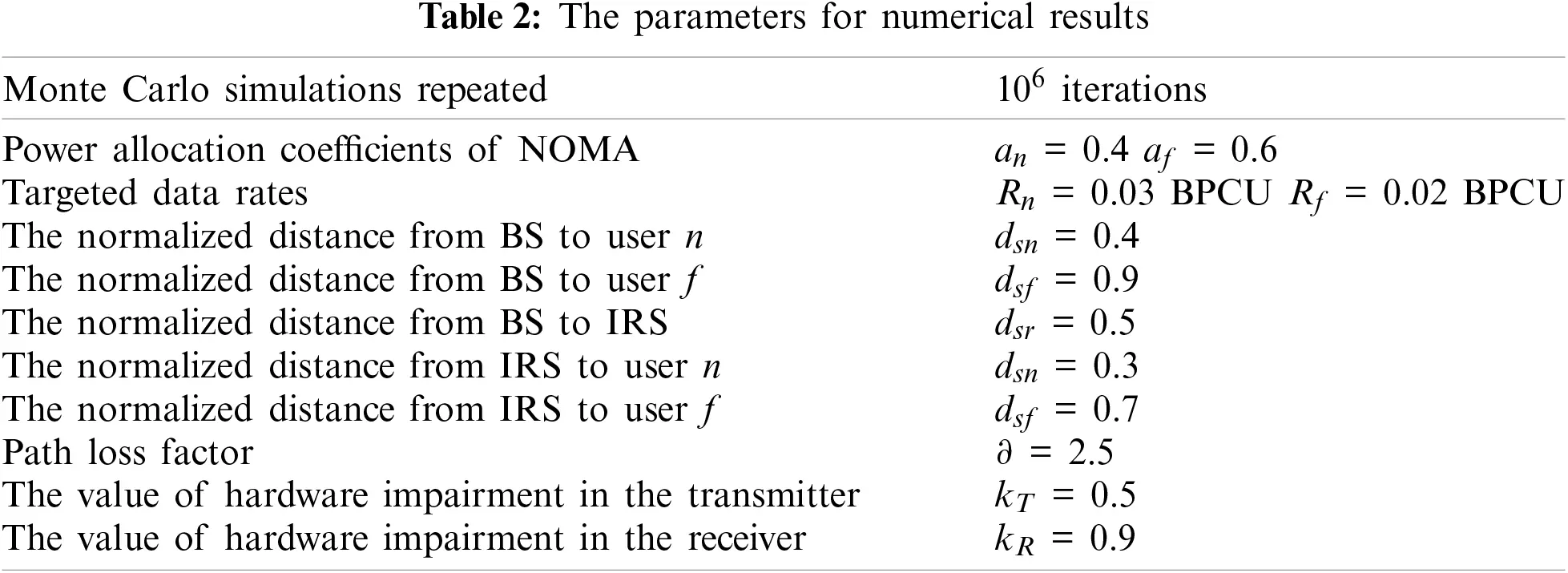Fig.2 plots the outage probability of two users for IRS-NOMA-HI networks vs. the transmit SNR, with M = 5, K = 5, E{|hI|2}=30 dB, Rn = 0.03 and Rf = 0.02 BPCU. For comparison, the outage performance of the traditional IRS-OMA-HI schemes is presented as well. The exact black and red solid curves for the outage probability of user n with pSIC/ipSIC are plotted according to (11) and (12), respectively. The blue dotted curve for asymptotic outage probability of user n according to (18) and (19), respectively. Furthermore, this figure depicts the outage probability of user f in (14) vs. the SNR obtained from the approximate expression in (20) and Monte-Carlo simulations from (13). The exact outage probability cures for the IRS-OMA-HI scheme are plotted according to (16). The simulation is identical to analytical curves across the entire SNR range, which verifies the outage performance. As a benchmark, the outage behavior for IRS-OMA-HI shows the worst performance. The reason is that NOMA differs from conventional OMA by using non-orthogonal communication at the transmitter side and achieving correct demodulation at the receiver side utilizing SIC techniques. The IRS-NOMA-HI has been able to provide higher user fairness relative to IRS-OMA-HI. One can observe that the outage probability with ipSIC converges to an error floor in the high SNR region and obtain a zero diversity order. This is due to the fact that the residual interference from ipSIC for IRS-NOMA-HI, which is also confirmed in Remark 1. Another observation is that the outage behavior of user n with pSIC outperforms that of user n with ipSIC and user f for IRS-NOMA-HI networks. This is due to the fact that the user n with pSIC has access to a larger diversity order than user f, in line with the insights of Remark 2. Due to residual interference, the outage probability of user n with ipSIC converges to an error floor and the diversity gain is zero. It can also be observed that the outage performance of user n with ipSIC gets worse as the residual interference value increases. In addition, the outage performance of user n can be improved in the high SNR region, although this user has HI.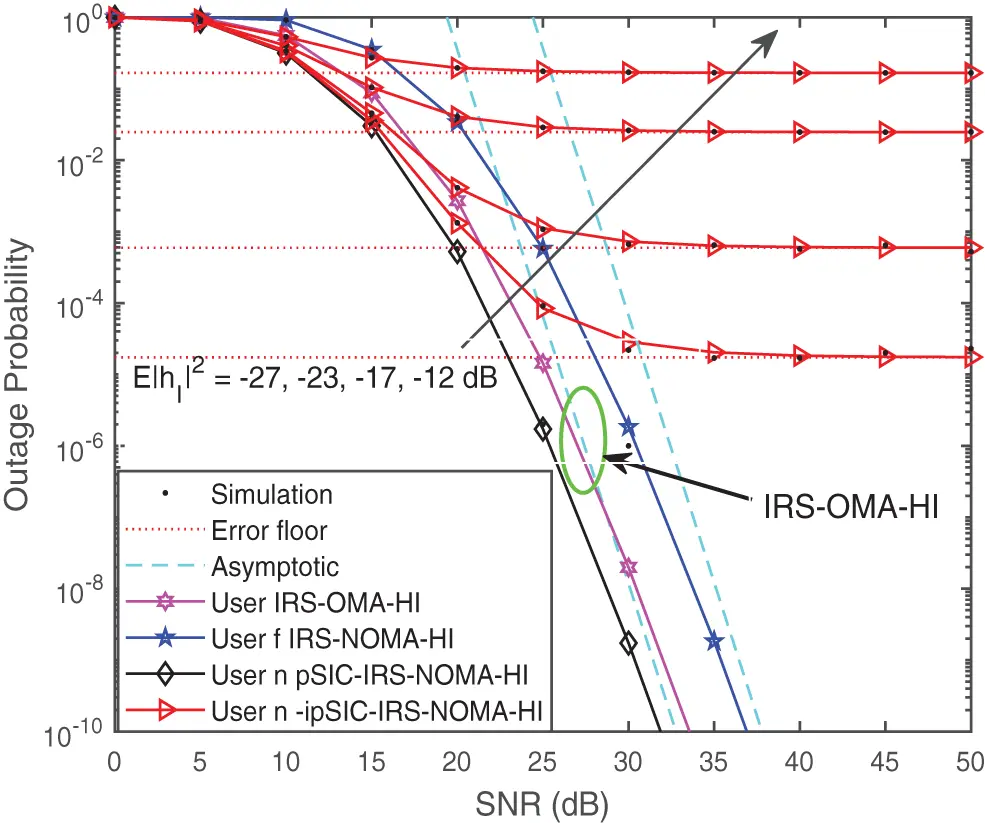Figure 2: Outage probability vs. the transmit SNR, with Rf = 0.03, Rn = 0.02 BPCU, M = 5, K = 5 and E{|hI|2}=5 dB

Fig.3 illustrates the outage probability of two users for IRS-NOMA-HI networks vs. the transmit SNR, for a simulation setting with K = 5, Rn = 0.03 and Rf = 0.02 BPCU. For high values of the number of IRS elements, the outage probability for IRS-NOMA-HI gains a steeper slope and achieves enhanced outage performance. It is noted that the diversity order found in Remark 2 and Remark 3 depends on the number of IRS elements and the values of HI. This phenomenon indicates the promising integration of IRS technologies in cooperative NOMA communications. Fig.4 shows the outage probability of two users for IRS-NOMA-HI networks vs. the transmit SNR with different values of target rate. More specifically, the values of Rn are increased from 0.02 BPCU to 0.16 BPCU, and the values of Rf are increased from 0.01 BPCU to 0.12 BPCU. It can be observed that the outage probability decreases with the increase of target rate values.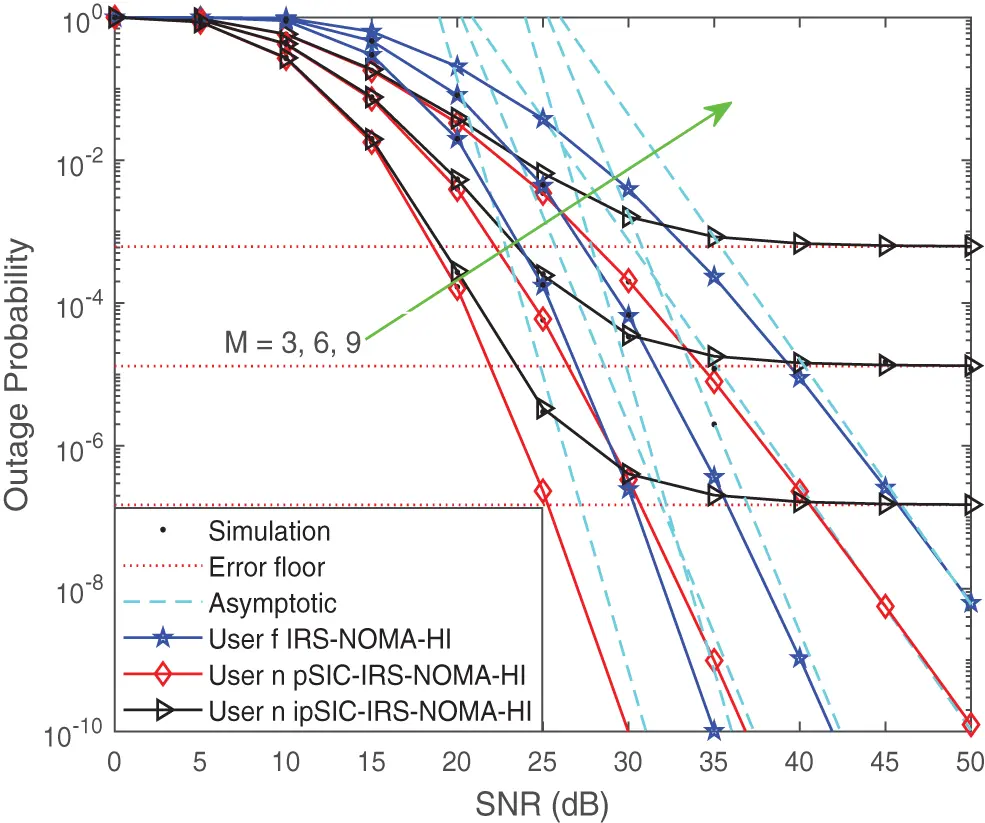Figure 3: Outage probability vs. the transmit SNR, with the different M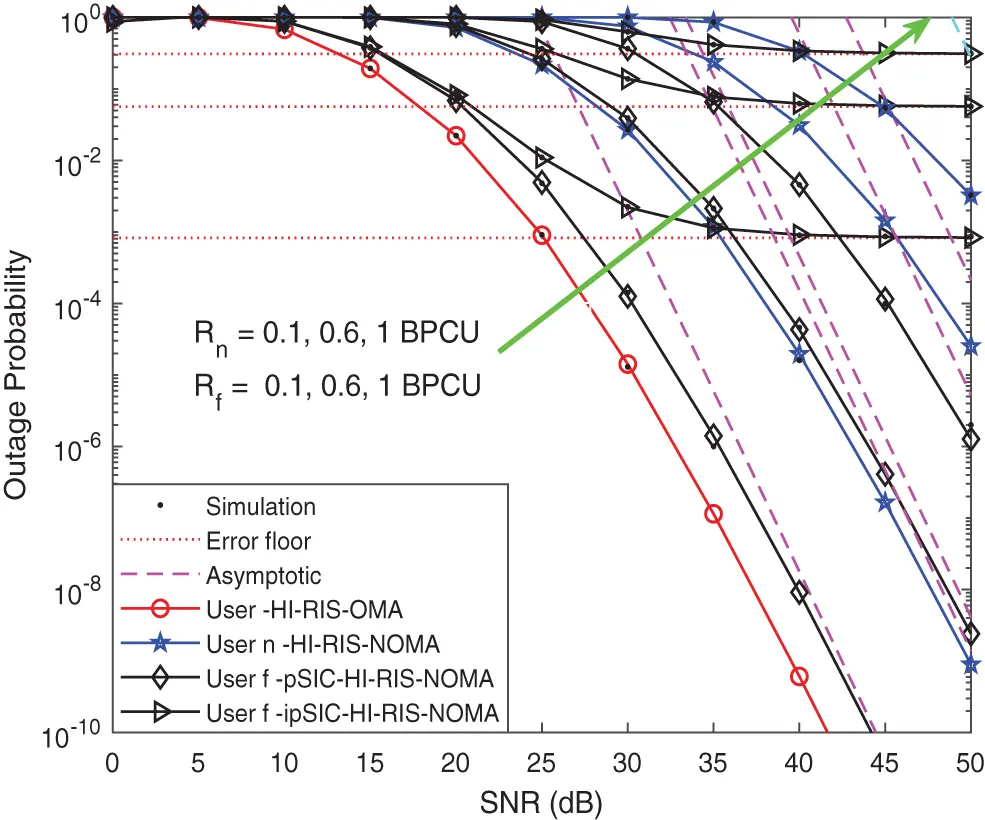Figure 4: Outage probability vs. the transmit SNR, with the different target rates

Fig. 5 plots the outage probability of two users for IRS-NOMA-HI networks vs. the transmit SNR, with M = 5, K = 5 and E{|hI|2}=30 dB. It can be seen that as the Rician factor decreases, the IRS-NOMA-HI network is capable of achieving enhanced outage performance. This phenomenon can be explained by the larger the Rician factor K, the more significant the impact on channel capacity and the increasing damage to the IRS-NOMA-HI network, especially as the SNR increases. As a further advance, Fig. 6 depicts the outage probability vs. the transmit SNR, with the different values of pass loss factors. We can observe the influence of the values of pass loss factors on the performance of IRS-NOMA-HI; the higher the values are, the better the performance of the outage probability is. This is due to the fact that the path loss is determined by the radiated spread of the transmit power and the propagation characteristics of the channel, and the channels are mainly influenced by line-of-sight component when the Rician factor is large.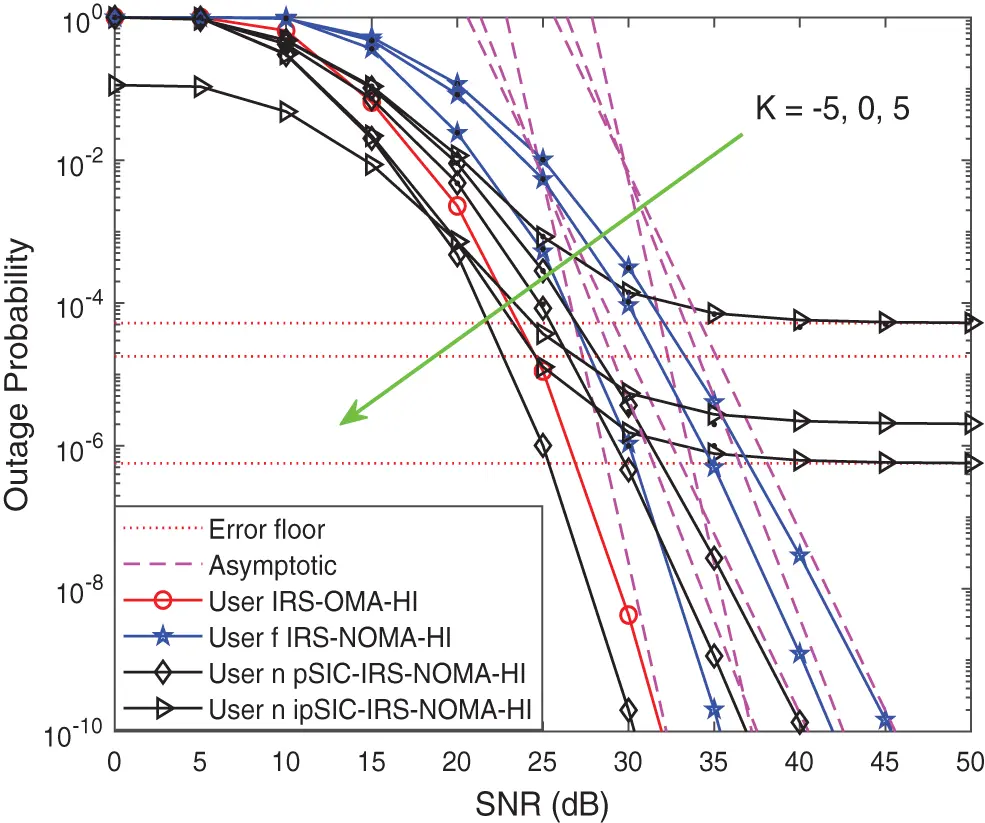Figure 5: Outage probability vs. the transmit SNR, with the different Rician factors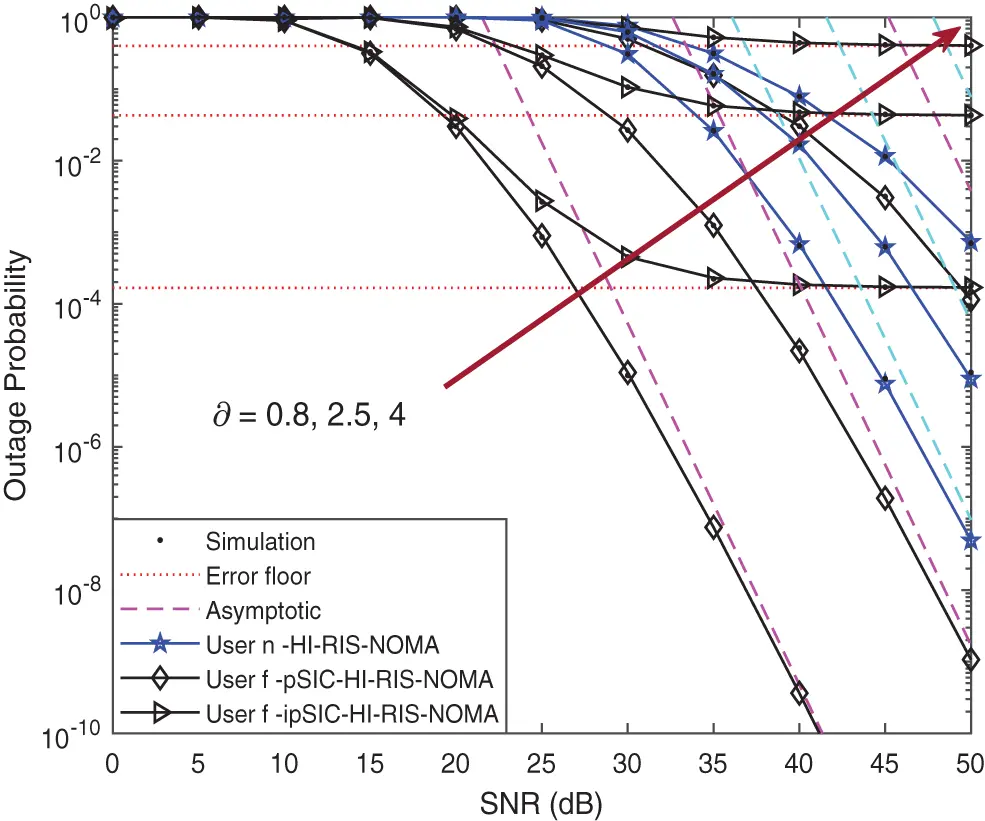Figure 6: Outage probability vs. the transmit SNR, with the different pass loss factors

Fig. 7 plots the outage probability of two users for IRS-NOMA-HI networks vs. the transmit SNR, with M = 5, K = 5 and E{|hI|2}=30 dB. It can be observed from the figure that the bad impact of IRS-NOMA-HI networks becomes more severe than the value of HI. The perfect value of HI kT = kR = 0 is shown as a benchmark, and the best outage performance can be observed for the ideal case in which both the transmitter and receiver do not experience the impact of HI. It is worth noting the importance of accurately modeling HI at the transmitter and receivers when evaluating the performance of IRS-NOMA-HI networks.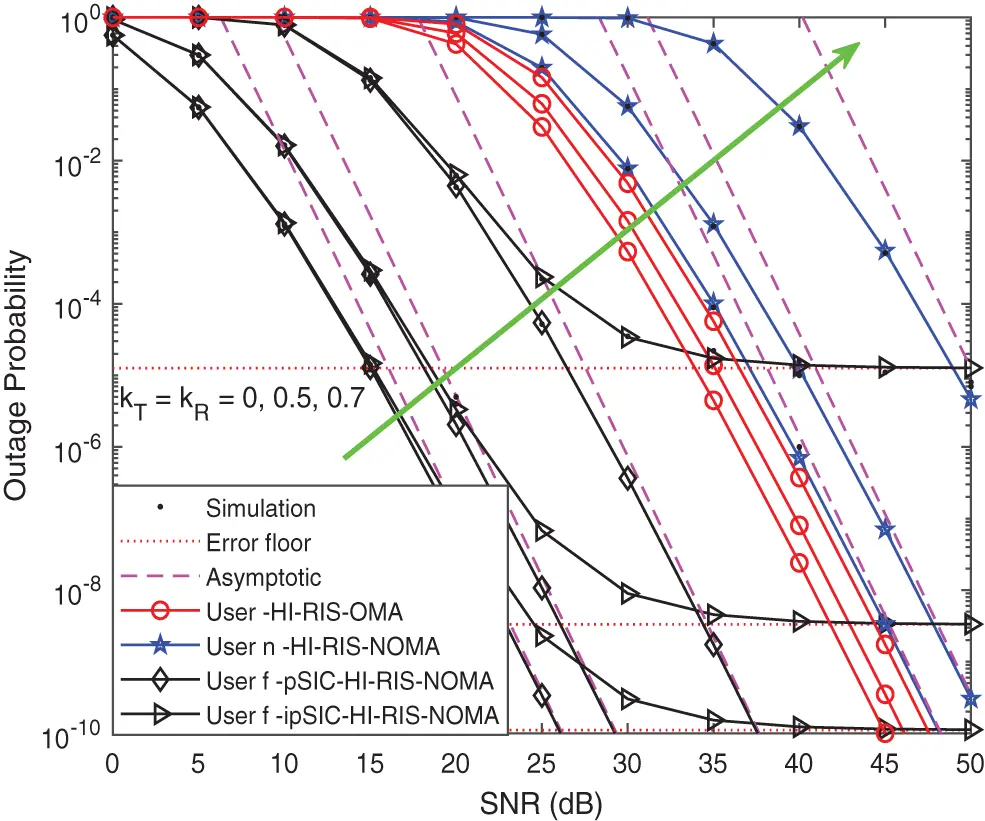Figure 7: Outage probability vs. the transmit SNR, with the different value of HI

Fig. 8 plots the curve of system throughput vs. the transmit SNR in delay-limited transmission mode, for a simulation setting with Rn = 1, Rf = 1 BPCU, M = 5 and K = 5. In this figure, the curves of system throughput with the transmit SNR in IRS-OMA-HI and IRS-NOMA-HI schemes are drawn according to (21). It can be observed from the figure that the system throughput for IRS-NOMA-HI in delay-limited transmission mode is better than that of IRS-OMA-HI. It can be explained that the system throughput depends on achieved the outage probability of users. This finding facilitates the design of IRS in wireless systems to cater to mass connections.Figure 8: System throughput vs. the transmit SNR in delay-limited transmission mode

5  Conclusion

Although NOMA communication can raise the outage performance and capacity of the traditional OMA wireless system, the residual interference generated by ipSIC and HI imposes a detrimental effect on system performance. This paper examined the joint impact of HI and residual interference on the outage probability and system throughput for the IRS-NOMA-HI and IRS-OMA-HI networks over Rician channels. In particular, we derived the exact and asymptotic expressions of outage probability in both pSIC and ipSIC cases and analyzed the diversity orders to evaluate their performance under various scenarios. Based on the numerical results, it illustrated that the outage performance and system throughput of IRS-NOMA-HI outperformed that of IRS-OMA-HI networks, which can be a promising candidate for future systems. It is recommended that more efforts should be made to suppress the effects of HI and residual interference when deploying IRS-NOMA-HI networks in realistic scenarios.

Funding Statement: This work was supported by the National Natural Science Foundation of China under Grants 62071052, 61901043 and the R&D Program of Beijing Municipal Education Commission under Grant KM202011232003. The work of W. Tang was supported by Scientific Research Projects supported by Talent Engineering Training Funds of Hebei Province under Grant A202101106 and Science and Technology Project of Hebei Education Department under Grant QN2020508.

Conflicts of Interest: The authors declare that they have no conflicts of interest to report regarding the present study.

1Note that multiple antennas equipped by the BS and users will further suppress the self-interference and enhance the performance of the IRS-NOMA networks, which are set aside for our future work.

2It is worth noting that the nearby user and distant user are distinguished based on the distance from the users to BS. For example, user n are near to BS, while user f are far away from BS.

## References

1. Liu, Y., Qin, Z., Elkashlan, M., Nallanathan, A., & McCann, J. A. (2017). Non-orthogonal multiple access in large-scale heterogeneous networks. IEEE Journal on Selected Areas in Communications, 35(12), 2667-2680. [Google Scholar] [CrossRef]
2. Liu, Y., Qin, Z., Elkashlan, M., Ding, Z., Nallanathan, A. et al. (2017). Non-orthogonal multiple access for 5G and beyond. Proceedings of the IEEE, 105(12), 2347–2381.
3. Ding, Z., Yang, Z., Fan, P., & Poor, H. V. (2014). On the performance of non-orthogonal multiple access in 5G systems with randomly deployed users. IEEE Signal Processing Letters, 21(12), 1501-1505. [Google Scholar] [CrossRef]
4. Jee, A., Janghel, K., & Prakriya, S. (2022). Performance of adaptive multi-user underlay NOMA transmission with simple user selection. IEEE Transactions on Cognitive Communications and Networking, 8(2), 871-887. [Google Scholar] [CrossRef]
5. Chen, X., Jia, R., & Ng, D. W. K. (2018). On the design of massive non-orthogonal multiple access with imperfect successive interference cancellation. IEEE Transactions on Communications, 67(3), 2539-2551. [Google Scholar] [CrossRef]
6. Gui, G., Sari, H., & Biglieri, E. (2019). A new definition of fairness for non-orthogonal multiple access. IEEE Communications Letters, 23(7), 1267-1271. [Google Scholar] [CrossRef]
7. Ding, Z., Peng, M., & Poor, H. V. (2015). Cooperative non-orthogonal multiple access in 5G systems. IEEE Communications Letters, 19(8), 1462-1465. [Google Scholar] [CrossRef]
8. Zhong, C., & Zhang, Z. (2016). Non-orthogonal multiple access with cooperative full-duplex relaying. IEEE Communications Letters, 20(12), 2478-2481. [Google Scholar] [CrossRef]
9. Yue, X., Liu, Y., Kang, S., Nallanathan, A., & Ding, Z. (2017). Exploiting full/half-duplex user relaying in NOMA systems. IEEE Transactions on Communications, 66(2), 560-575. [Google Scholar]
10. Fan, L., Zhao, N., Lei, X., Chen, Q., & Yang, N. (2018). Outage probability and optimal cache placement for multiple amplify-and-forward relay networks. IEEE Transactions on Vehicular Technology, 67(12), 12373-12378. [Google Scholar] [CrossRef]
11. Jee, A., Agrawal, K., & Prakriya, S. (2021). A coordinated direct AF/DF relay-aided NOMA framework for low outage. IEEE Transactions on Communications, 70(3), 1559-1579. [Google Scholar]
12. Pan, C., Ren, H., Wang, K., Kolb, J. F., & Elkashlan, M. (2021). Reconfigurable intelligent surfaces for 6G systems: Principles, applications, and research directions. IEEE Communications Magazine, 59(6), 14-20. [Google Scholar] [CrossRef]
13. Chowdhury, M. Z., Shahjalal, M., Ahmed, S., & Jang, Y. M. (2020). 6G wireless communication systems: Applications, requirements, technologies, challenges, and research directions. IEEE Open Journal of the Communications Society, 1, 957-975. [Google Scholar] [CrossRef]
14. ElMossallamy, M. A., Zhang, H., Song, L., Seddik, K. G., & Han, Z. (2020). Reconfigurable intelligent surfaces for wireless communications: Principles, challenges, and opportunities. IEEE Transactions on Cognitive Communications and Networking, 6(3), 990-1002. [Google Scholar] [CrossRef]
15. di Renzo, M., Zappone, A., Debbah, M., Alouini, M. S., & Yuen, C. (2020). Smart radio environments empowered by reconfigurable intelligent surfaces: How it works, state of research, and the road ahead. IEEE Journal on Selected Areas in Communications, 38(11), 2450-2525. [Google Scholar] [CrossRef]
16. Wu, Q., & Zhang, R. (2020). Towards smart and reconfigurable environment: Intelligent reflecting surface aided wireless network. IEEE Communications Magazine, 58(1), 106-112. [Google Scholar] [CrossRef]
17. Yu, X., Xu, D., Sun, Y., Ng, D. W. K., & Schober, R. (2020). Robust and secure wireless communications via intelligent reflecting surfaces. IEEE Journal on Selected Areas in Communications, 38(11), 2637-2652. [Google Scholar] [CrossRef]
18. Shen, H., Xu, W., Gong, S., He, Z., & Zhao, C. (2019). Secrecy rate maximization for intelligent reflecting surface assisted multi-antenna communications. IEEE Communications Letters, 23(9), 1488-1492. [Google Scholar] [CrossRef]
19. Huang, C., Zappone, A., Alexandropoulos, G. C., Debbah, M., & Yuen, C. (2019). Reconfigurable intelligent surfaces for energy efficiency in wireless communication. IEEE Transactions on Wireless Communications, 18(8), 4157-4170. [Google Scholar] [CrossRef]
20. Bariah, L., Muhaidat, S., Sofotasios, P. C., El Bouanani, F., & Dobre, O. A. (2021). Large intelligent surface-assisted nonorthogonal multiple access for 6G networks: Performance analysis. IEEE Internet of Things Journal, 8(7), 5129-5140. [Google Scholar] [CrossRef]
21. Wang, H., Liu, C., Shi, Z., Fu, Y., & Song, R. (2020). On power minimization for IRS-aided downlink NOMA systems. IEEE Wireless Communications Letters, 9(11), 1808-1811. [Google Scholar] [CrossRef]
22. Mu, X., Liu, Y., Guo, L., Lin, J., & Al-Dhahir, N. (2020). Exploiting intelligent reflecting surfaces in NOMA networks: Joint beamforming optimization. IEEE Transactions on Wireless Communications, 19(10), 6884-6898. [Google Scholar] [CrossRef]
23. Hou, T., Liu, Y., Song, Z., Sun, X., & Chen, Y. (2020). Reconfigurable intelligent surface aided NOMA networks. IEEE Journal on Selected Areas in Communications, 38(11), 2575-2588. [Google Scholar] [CrossRef]
24. Fang, F., Xu, Y., Pham, Q. V., & Ding, Z. (2020). Energy-efficient design of IRS-NOMA networks. IEEE Transactions on Vehicular Technology, 69(11), 14088-14092. [Google Scholar] [CrossRef]
25. Cheng, Y., Li, K. H., Liu, Y., Teh, K. C., & Poor, H. V. (2021). Downlink and uplink intelligent reflecting surface aided networks: NOMA and OMA. IEEE Transactions on Wireless Communications, 20(6), 3988-4000. [Google Scholar] [CrossRef]
26. Yue, X., & Liu, Y. (2022). Performance analysis of intelligent reflecting surface assisted NOMA networks. IEEE Transactions on Wireless Communications, 21(4), 2623-2636. [Google Scholar] [CrossRef]
27. Ding, Z., & Poor, H. V. (2020). A simple design of IRS-NOMA transmission. IEEE Communications Letters, 24(5), 1119-1123. [Google Scholar] [CrossRef]
28. Fu, M., Zhou, Y., Shi, Y. (2019). Intelligent reflecting surface for downlink non-orthogonal multiple access networks. 2019 IEEE Globecom Workshops (GC Wkshps), pp. 1–6. Hawaii.
29. Liu, X., Liu, Y., & Chen, Y. (2020). Machine learning empowered trajectory and passive beamforming design in UAV-RIS wireless networks. IEEE Journal on Selected Areas in Communications, 39(7), 2042-2055. [Google Scholar] [CrossRef]
30. Zhong, R., Liu, Y., Mu, X., Chen, Y., & Song, L. (2021). AI empowered RIS-assisted NOMA networks: Deep learning or reinforcement learning?. IEEE Journal on Selected Areas in Communications, 40(1), 182-196. [Google Scholar] [CrossRef]
31. Li, Z., Chen, M., Yang, Z., Zhao, J., & Wang, Y. (2021). Energy efficient reconfigurable intelligent surface enabled mobile edge computing networks with NOMA. IEEE Transactions on Cognitive Communications and Networking, 7(2), 427-440. [Google Scholar] [CrossRef]
32. Zhang, Z., Zhang, C., Jiang, C., Jia, F., & Ge, J. (2021). Improving physical layer security for reconfigurable intelligent surface aided NOMA 6G networks. IEEE Transactions on Vehicular Technology, 70(5), 4451-4463. [Google Scholar] [CrossRef]
33. Li, X., Li, J., Liu, Y., Ding, Z., & Nallanathan, A. (2019). Residual transceiver hardware impairments on cooperative NOMA networks. IEEE Transactions on Wireless Communications, 19(1), 680-695. [Google Scholar] [CrossRef]
34. Li, X., Li, J., & Li, L. (2019). Performance analysis of impaired SWIPT NOMA relaying networks over imperfect weibull channels. IEEE Systems Journal, 14(1), 669-672. [Google Scholar] [CrossRef]
35. Bjornson, E., Matthaiou, M., & Debbah, M. (2013). A new look at dual-hop relaying: Performance limits with hardware impairments. IEEE Transactions on Communications, 61(11), 4512-4525. [Google Scholar] [CrossRef]
36. Li, X., Zhao, M., Zeng, M., Mumtaz, S., & Menon, V. G. (2021). Hardware impaired ambient backscatter NOMA systems: Reliability and security. IEEE Transactions on Communications, 69(4), 2723-2736. [Google Scholar] [CrossRef]
37. Deng, C., Liu, M., Li, X., & Liu, Y. (2020). Hardware impairments aware full-duplex NOMA networks over rician fading channels. IEEE Systems Journal, 15(2), 2515-2518. [Google Scholar] [CrossRef]
38. Li, X., Wang, Q., Liu, M., Li, J., & Peng, H. (2020). Cooperative wireless-powered NOMA relaying for B5G IoT networks with hardware impairments and channel estimation errors. IEEE Internet of Things Journal, 8(7), 5453-5467. [Google Scholar] [CrossRef]
39. Zhou, G., Pan, C., Ren, H., Wang, K., & Peng, Z. (2021). Secure wireless communication in RIS-aided MISO system with hardware impairments. IEEE Wireless Communications Letters, 10(6), 1309-1313. [Google Scholar] [CrossRef]
40. Papazafeiropoulos, A., Sharma, S. K., Ratnarajah, T., & Chatzinotas, S. (2017). Impact of residual additive transceiver hardware impairments on Rayleigh-product mimo channels with linear receivers: Exact and asymptotic analyses. IEEE Transactions on Communications, 66(1), 105-118. [Google Scholar] [CrossRef]
41. Hemanth, A., Umamaheswari, K., Pogaku, A. C., Do, D. T., & Lee, B. M. (2020). Outage performance analysis of reconfigurable intelligent surfaces-aided NOMA under presence of hardware impairment. IEEE Access, 8, 212156-212165. [Google Scholar] [CrossRef]
42. Chen, Q., Li, M., Yang, X., Alturki, R., & Alshehri, M. D. (2021). Impact of residual hardware impairment on the IoT secrecy performance of RIS-assisted NOMA networks. IEEE Access, 9, 42583-42592. [Google Scholar] [CrossRef]
43. Saeidi, M. A., Emadi, M. J., Masoumi, H., Mili, M. R., & Ng, D. W. K. (2021). Weighted sum-rate maximization for multi-IRS-assisted full-duplex systems with hardware impairments. IEEE Transactions on Cognitive Communications and Networking, 7(2), 466-481. [Google Scholar] [CrossRef]
44. Zhang, J., Zhong, C., & Zhang, Z. (2022). An efficient calibration algorithm for IRS-Aided mmWave systems with hardware impairments. IEEE Communications Letters, 26(1), 172-176. [Google Scholar] [CrossRef]
45. Liu, Y., Qin, Z., Elkashlan, M., Gao, Y., & Hanzo, L. (2017). Enhancing the physical layer security of non-orthogonal multiple access in large-scale networks. IEEE Transactions on Wireless Communications, 16(3), 1656-1672. [Google Scholar] [CrossRef]
46. Hildebrand, E. (1987). Introduction to numerical analysis. New York, NY, USA: Dover.

Appendix A. Proof of Theorem 1

According to the definition of outage probability, the outage probability of user n for IRS-NOMA-HI networks can be expressed by

Pn=Pr(γnf<γthf)+Pr(γnf>γthf,γn<γthf)=Pr[ 0<| hsn+hsrHΦhrn |2<β(ϖ| hI |2ρs+1) ], (A.1)

where β=γthnρs[anγthn(kT2+kR2)]. Upon substituting f|hI|2(y)=1ΩIeyΩI into (11) and using the CDF of Z, the outage probability of user n with ipSIC can be given by

PnipSIC=0f| hI |2(y)FZ[ β(ϖ| hI |2ρs+1) ]dy

=00β(ϖyρs+1)1ϕΓ(φ+1)ΩI(xϕ)φexp[ xϕyΩI ]×{ 1Q(2K,[ β(ϖyρs+1)x ]2(K+1)αsn) }dxdy. (A.2)

By using the Gauss-Chebyshev quadrature , the complex definite integral in the above equation can be approximated as

PnipSICΥl=1L(1xl2)(xl+1)φ0νφ+1exp[ (xl+1)ν2ϕx ]×{ 1Q(2K,[ (1xl)ν2 ]2(K+1)αsn) }dx, (A.3)

where ν=β(ϖΩIxρs+1), Υ=π(2ϕ)φ+1LΓ(φ+1) and xl=cos(2l12Lπ). Upon substituting y=ΩIx into the above equation and using the Gauss-Chebyshev quadrature, the outage probability of user n with ipSIC can be further obtained. The proof is completed.

Appendix B. Proof of Theorem 2

Base on (13), the outage probability of user f for IRS-NOMA-HI networks can be rewritten as

Pf=Pr(| hsf+hsrHΦhrf |2<γthfρs(afγthfan(kT2+kR2)))=Pr(| | hsf |+m=1M| hsrmhrfm | |2Z<α), (B.1)

where α=γthfρs(afγthfan(kT2+kR2)). Upon substituting (10) into (B.1), the outage probability of user f can be further expressed as

Pf=2(K+1)Γ(φ+1)αsfeK0αexp[(K+1)x2αsf]xK0(2xK(K+1)αsf)γ(φ+1,αxϕ)dx. (B.2)

By using the Gauss-Chebyshev quadrature, the outage probability of user n for IRS-NOMA-HI networks can be finally expressed as

Pfl=1Lπα(K+1)2LαsfeKΓ(φ+1)exp(α(K+1)(xl+1)24αsf)AlK0((xl+1)αK(K+1)αsf)γ(φ+1,ααxl2ϕ). (B.3)

Liu, Z., Yue, X., Chen, S., Liu, X., Wang, Y. et al. (2023). Exploiting the Direct Link in IRS Assisted NOMA Networks with Hardware Impairments. CMES-Computer Modeling in Engineering & Sciences, 136(1), 767–785.This work is licensed under a Creative Commons Attribution 4.0 International License , which permits unrestricted use, distribution, and reproduction in any medium, provided the original work is properly cited.

View

Like

## Related articles

• Shanshan Yu, Ju Liu, Xiaoqing...
• Yinghua Zhang, Yanfang Dong, Lei...
• Lei Wang, Jian Liu, Alan Yang
• Ruoyu Su, Xiaojun Sun, Fei Ding,...
• Jian Liu, Lei Wang, Changming...